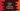# Python program to print Arithmetic progression series## Python program to print Arithmetic progression:

In this post, we will write one python program that will print the Arithmetic progression series or AP series. This program will take the values of starting number, common difference, and total numbers as inputs from the user and print the series starting from the given number.

Let’s quickly learn what is Arithmetic progression and how it looks like:

### What is Arithmetic progression:

Arithmetic progression or AP or Arithmetic equence is a sequence of numbers with difference between each numbers is constant. This constant value is called common difference.

For example, 1, 3, 5, 7, 9, 11… is an Arithmetic progression with common difference 2.

This is an infinite series of numbers and we want to print it for a specific number of times. So, in this program, we will take this value from the user.

## Algorithm to follow:

We will use the below algorithm to print the Arithmetic progression.

• Take the value of start element, common difference and total number of items to print from the user.
• Initialize one variable and assign the value of start element to this variable. This variable will be used to store the current value in the series.
• Run a loop for n number of times, where n is the user given total number of items to print.

• On each iteration, print the current value of the Arithmetic progression, i.e. the variable initialized in the second step.
• Add common difference to the current value variable.
• Move to next iteration.

## Python program:

Now, let’s write down the program:

``````def print_arithmetic_progression(a, d, n):
current_value = a

for i in range(0, n):
print(current_value, end=' ')
current_value = current_value + d

a = int(input('Enter the start number: '))
d = int(input('Enter the common difference: '))
n = int(input('Enter total numbers to print: '))
print_arithmetic_progression(a, d, n)``````

Here,

• This program reads the start number, common difference and total numbers values and stores these in a, d and n.
• printarithmeticprogression method is used to print the Arithmetic progression series.
• current_value variable is initialized as a, which is the current value to print.
• The for loop prints the current value of the series and increments it by adding common difference to it.

If you run this program, it will print the below output:

``````Enter the start number: 2
Enter the common difference: 3
Enter total numbers to print: 5
2 5 8 11 14

Enter the start number: 5
Enter the common difference: 5
Enter total numbers to print: 5
5 10 15 20 25``````

You can also use any other loop if you want.